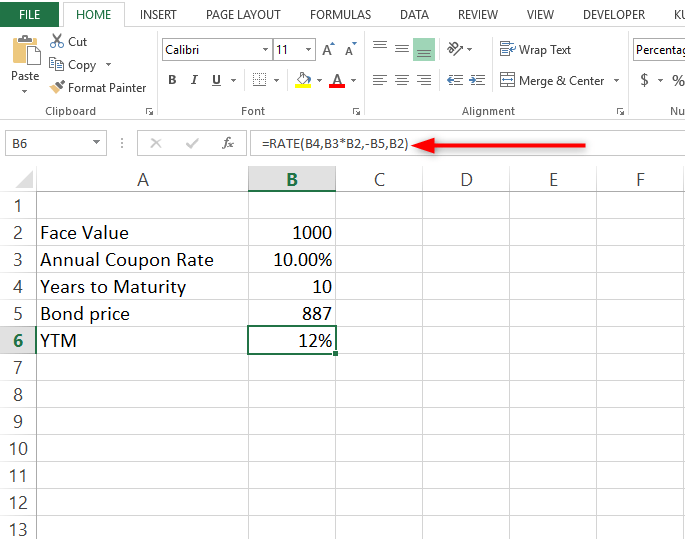# How to calculate YTM in Excel

YTM means yield to maturity. It is also known as the internal rate of return. If you are dealing with finances, this is one of the values you have to calculate when dealing with compound interest. For you to calculate YTM using Excel formulas, there are some values you need to have. These include the initial principal amount invested, the interest rate to be paid yearly, the time duration the principal amount has been invested, and the rate of daily, monthly or quarterly payments. With all these values available, Excel will calculate for you the YTM value using the RATE function.

This article gives you step-by-step instructions on calculating YTM in Excel workbooks using the bond yield calculator.

## Steps to follow when calculating YTM in Excel using =RATE ()

Let us use these values for this example. You can replace them with your values. Face value =1000 Annual coupon rate =10% Years to maturity =10 Bond price =887. Now let us create the YTM using these values.

1. Launch the Microsoft Excel program on your computer.2. Write the following words from cells A2 –A5. Future Value, Annual Coupon rate, Years to maturity, and Bond Price3. Format the column width in the excel sheet so that it is wide enough to accommodate all characters.

4. Let us enter the corresponding values of our example in Column B. You can replace these values with your own.5. Write YTM in cell A6.6. Now, this is the crucial part. In the corresponding cell, B6 type the following formula =RATE(B4,B3*B2,-B5,B2) Press enter and the answer is the Yield to Maturity rate in %.## Using the Excel IRR Function

This function’s syntax is as follows

IRR (values, [guess])

It is broken down as follows;

IRR is the internal rate of return for a certain period. For instance, if it is calculated semi-annually, you can double the result to give you the annual bond returns.

Values represent the future bond cash flows. Values must be denoted with a negative and positive value. For instance, say the bond payment was \$1000. This shall be written as -\$1000 at the start of the payment period. At bond maturity, we shall be able to then calculate the period’s payment coupon and cash flow for future bonds.

Guess this value represents an arbitrary value (a guess value) of what your internal rate of return can be. Using this value is optional. for more lessons on how to calculate the internal rate of returns, click here.I inputted random numbers in the above template and you can see the values are tabulated at the end.  Therefore, with company information, you can easily calculate yields to maturity by inputting values in a template like the one above in excel.

## Uses of YTM

It is important to understand YTM as it is used to assess expected performance. The golden principle here is that the expected bond return rate changes depending on the market price despite the constant coupon rate. It is used to reflect how favorable the market is for the bond. Hence it is vital for people to manage a bond investment portfolio.

The formula for pricing a bond

You can price a bond by using the following formula

PV = Payment / (1+r)+ Payment / (1+r)+ ..+ Payment + Principle / (1+r)

Syntax derivatives

Pv = Price of the bond

Payment =Also referred to as the coupon payment. This is the coupon rate * par value ÷ number of payments per year

r stands for the required rate of return. It's actually the required rate of return divided by the number of payments per year.

N= Years until maturity

Principal= face value of the bond/par value

As you can see from the above formula the price of the bond depends on the difference between the coupon rate and inferred required rate and

## Conclusion

The steps above are the manual way of calculating YTM in an Excel worksheet. It is a straightforward and easy process to accomplish once you know your values and the formula to apply.

In case you find it a challenge using the above method, you can calculate YTM using the RATE and IRR functions in Excel.